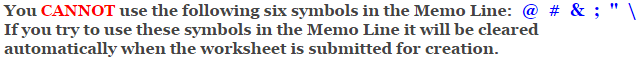# Calculus

## Differential Equation Slope Field Worksheets

These Calculus Worksheets will produce problems that deal with using a slope field to graph a differential equation.
The student will be given differential equations and slope fields, and will be asked to graph the antiderivative of the equation.
You may select the number of problems, the types of equations to use, and the notation.

These Differential Equation Slope Field Worksheets are a great resource for Differentiation Applications.

### Language for the Differential Equation Slope Field Worksheets

 English German Albanian Spanish Swedish Italian French Turkish Polish Norwegian

### Memo Line for the Differential Equation Slope Field Worksheets

You may enter a message or special instruction that will appear on the bottom left corner of the Differential Equation Slope Field Worksheets.### Differential Equation Slope Field Worksheets Answer Page

Now you are ready to create your Differential Equation Slope Field Worksheets by pressing the Create Button.

 Recommended Videos

If You Experience Display Problems with Your Math Worksheet